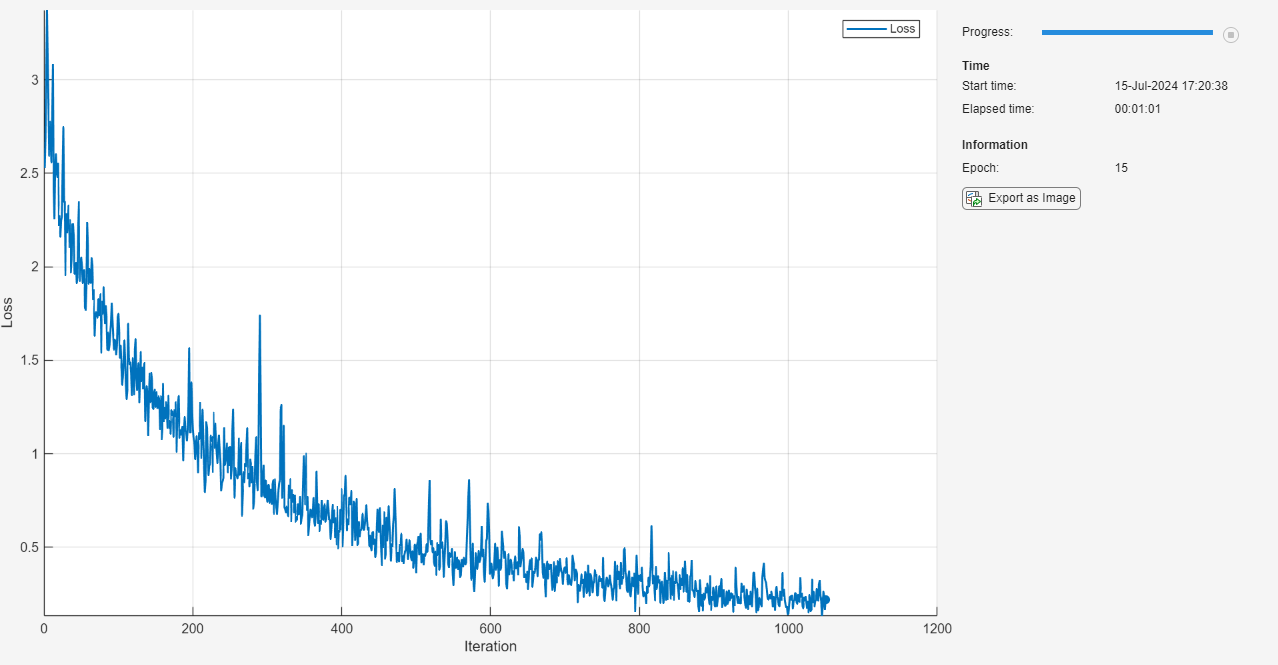Documentation

## Train Network Using Custom Training Loop

This example shows how to train a network that classifies handwritten digits with a custom learning rate schedule.

If `trainingOptions` does not provide the options you need (for example, a custom learning rate schedule), then you can define your own custom training loop using automatic differentiation.

This example trains a network to classify handwritten digits with the time-based decay learning rate schedule: for each iteration, the solver uses the learning rate given by ${\rho }_{\mathit{t}}=\frac{{\rho }_{0}}{1+\mathit{k}\text{\hspace{0.17em}}\mathit{t}}$, where t is the iteration number, ${\rho }_{0}$ is the initial learning rate, and k is the decay.

```[XTrain,YTrain] = digitTrain4DArrayData; classes = categories(YTrain); numClasses = numel(classes);```

### Define Network

Define the network and specify the average image using the `'Mean'` option in the image input layer.

```layers = [ imageInputLayer([28 28 1], 'Name', 'input', 'Mean', mean(XTrain,4)) convolution2dLayer(5, 20, 'Name', 'conv1') reluLayer('Name', 'relu1') convolution2dLayer(3, 20, 'Padding', 1, 'Name', 'conv2') reluLayer('Name', 'relu2') convolution2dLayer(3, 20, 'Padding', 1, 'Name', 'conv3') reluLayer('Name', 'relu3') fullyConnectedLayer(numClasses, 'Name', 'fc')]; lgraph = layerGraph(layers);```

Create a `dlnetwork` object from the layer graph.

`dlnet = dlnetwork(lgraph)`
```dlnet = dlnetwork with properties: Layers: [8×1 nnet.cnn.layer.Layer] Connections: [7×2 table] Learnables: [8×3 table] State: [0×3 table] ```

Create the function `modelGradients`, listed at the end of the example, that takes a `dlnetwork` object `dlnet`, a mini-batch of input data `dlX` with corresponding labels `Y` and returns the gradients of the loss with respect to the learnable parameters in `dlnet` and the corresponding loss.

### Specify Training Options

Specify the training options.

```velocity = []; numEpochs = 20; miniBatchSize = 128; numObservations = numel(YTrain); numIterationsPerEpoch = floor(numObservations./miniBatchSize); initialLearnRate = 0.01; momentum = 0.9; decay = 0.01;```

Train on a GPU if one is available. Using a GPU requires Parallel Computing Toolbox™ and a CUDA® enabled NVIDIA® GPU with compute capability 3.0 or higher.

`executionEnvironment = "auto";`

### Train Model

Train the model using a custom training loop.

For each epoch, shuffle the data and loop over mini-batches of data. At the end of each epoch, display the training progress.

For each mini-batch:

• Convert the labels to dummy variables.

• Convert the data to `dlarray` objects with underlying type single and specify the dimension labels `'SSCB'` (spatial, spatial, channel, batch).

• For GPU training, convert to `gpuArray` objects.

• Evaluate the model gradients and loss using `dlfeval` and the `modelGradients` function.

• Determine the learning rate for the time-based decay learning rate schedule.

• Update the network parameters using the `sgdmupdate` function.

Initialize the training progress plot.

```plots = "training-progress"; if plots == "training-progress" figure lineLossTrain = animatedline; xlabel("Iteration") ylabel("Loss") end```

Train the network.

```iteration = 0; start = tic; % Loop over epochs. for epoch = 1:numEpochs % Shuffle data. idx = randperm(numel(YTrain)); XTrain = XTrain(:,:,:,idx); YTrain = YTrain(idx); % Loop over mini-batches. for i = 1:numIterationsPerEpoch iteration = iteration + 1; % Read mini-batch of data and convert the labels to dummy % variables. idx = (i-1)*miniBatchSize+1:i*miniBatchSize; X = XTrain(:,:,:,idx); Y = zeros(numClasses, miniBatchSize, 'single'); for c = 1:numClasses Y(c,YTrain(idx)==classes(c)) = 1; end % Convert mini-batch of data to dlarray. dlX = dlarray(single(X),'SSCB'); % If training on a GPU, then convert data to gpuArray. if (executionEnvironment == "auto" && canUseGPU) || executionEnvironment == "gpu" dlX = gpuArray(dlX); end % Evaluate the model gradients and loss using dlfeval and the % modelGradients function. [gradients,loss] = dlfeval(@modelGradients,dlnet,dlX,Y); % Determine learning rate for time-based decay learning rate schedule. learnRate = initialLearnRate/(1 + decay*iteration); % Update the network parameters using the SGDM optimizer. [dlnet.Learnables, velocity] = sgdmupdate(dlnet.Learnables, gradients, velocity, learnRate, momentum); % Display the training progress. if plots == "training-progress" D = duration(0,0,toc(start),'Format','hh:mm:ss'); addpoints(lineLossTrain,iteration,double(gather(extractdata(loss)))) title("Epoch: " + epoch + ", Elapsed: " + string(D)) drawnow end end end```### Test Model

Test the classification accuracy of the model by comparing the predictions on a test set with the true labels.

`[XTest, YTest] = digitTest4DArrayData;`

Convert the data to a `dlarray` object with dimension format `'SSCB'`. For GPU prediction, also convert the data to `gpuArray`.

```dlXTest = dlarray(XTest,'SSCB'); if (executionEnvironment == "auto" && canUseGPU) || executionEnvironment == "gpu" dlXTest = gpuArray(dlXTest); end```

To classify images using a `dlnetwork` object, use the `predict` function and find the classes with the highest scores.

```dlYPred = predict(dlnet,dlXTest); [~,idx] = max(extractdata(dlYPred),[],1); YPred = classes(idx);```

Evaluate the classification accuracy.

`accuracy = mean(YPred==YTest)`
```accuracy = 0.9780 ```

The `modelGradients` function takes a `dlnetwork` object `dlnet`, a mini-batch of input data `dlX` with corresponding labels `Y` and returns the gradients of the loss with respect to the learnable parameters in `dlnet` and the corresponding loss. To compute the gradients automatically, use the `dlgradient` function.

```function [gradients,loss] = modelGradients(dlnet,dlX,Y) dlYPred = forward(dlnet,dlX); dlYPred = softmax(dlYPred); loss = crossentropy(dlYPred,Y); gradients = dlgradient(loss,dlnet.Learnables); end```